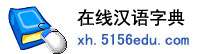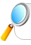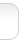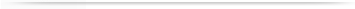汉字   按部首检索  按拼音检索王盛 陈盛 叶盛 李盛 张盛 徐盛 黄盛 丁盛 郭盛 周盛 林盛 杨盛 刘盛 赵盛 孙盛 吴盛 罗盛 强盛 唐盛 龚盛 汪盛 朱盛 常盛 谢盛 昌盛 章盛 许盛 邹盛 姜盛 夏盛

 盛字的男姓名 | 盛字的女姓名 | 盛开头的姓名 | 盛结尾的姓名 | 更多带盛的姓名
 盛 (男) 昌盛 (男) 茂盛 (男) 国盛 (男) 永盛 (男) 盛华 (男) 盛 (女) 盛林 (男) 文盛 (男) 德盛 (男) 兴盛 (男) 盛杰 (男) 盛强 (男) 春盛 (男) 盛昌 (男) 盛楠 (女) 华盛 (男) 盛军 (男) 盛龙 (男) 家盛 (男) 建盛 (男) 志盛 (男) 明盛 (男) 盛春 (男) 盛国 (男) 盛伟 (男) 盛荣 (男) 金盛 (男) 盛平 (男) 盛斌 (男) 盛辉 (男) 荣盛 (男) 盛华 (女) 盛文 (男) 盛东 (男) 盛梅 (女) 盛祥 (男) 福盛 (男) 光盛 (男) 盛峰 (男) 长盛 (男) 新盛 (男) 旺盛 (男) 树盛 (男) 鼎盛 (男) 宏盛 (男) 盛海 (男) 繁盛 (男) 盛波 (男) 继盛 (男) 盛勇 (男) 盛武 (男) 盛源 (男) 盛兰 (女) 盛旺 (男) 强盛 (男) 伟盛 (男) 盛凯 (男) 盛涛 (男) 盛楠 (男) 盛琦 (女) 盛发 (男) 盛德 (男) 盛阳 (男) 万盛 (男) 盛鑫 (男) 盛茂 (男) 盛威 (男) 立盛 (男) 盛敏 (男) 盛明 (男) 松盛 (男) 盛江 (男) 嘉盛 (男) 盛荣 (女) 彩盛 (男) 盛才 (男) 传盛 (男) 维盛 (男) 叶盛 (男) 盛春 (女) 盛艳 (女) 裕盛 (男) 盛男 (女) 友盛 (男) 盛超 (男) 林盛 (男) 富盛 (男) 盛民 (男) 盛达 (男) 世盛 (男) 锦盛 (男) 克盛 (男) 盛红 (男) 盛兴 (男) 庆盛 (男) 广盛 (男) 盛光 (男) 丰盛 (男) 祖盛 (男) 盛俊 (男) 盛兵 (男) 学盛 (男) 东盛 (男) 柏盛 (男) 正盛 (男) 盛盛 (男) 盛全 (男) 盛浩 (男) 盛财 (男) 盛飞 (男) 盛良 (男) 振盛 (男) 盛权 (男) 盛英 (女) 盛芳 (女) 盛琼 (女) 盛锋 (男) 盛松 (男) 盛刚 (男)

 版权所有　在线汉语字典   浙ICP备05019169号